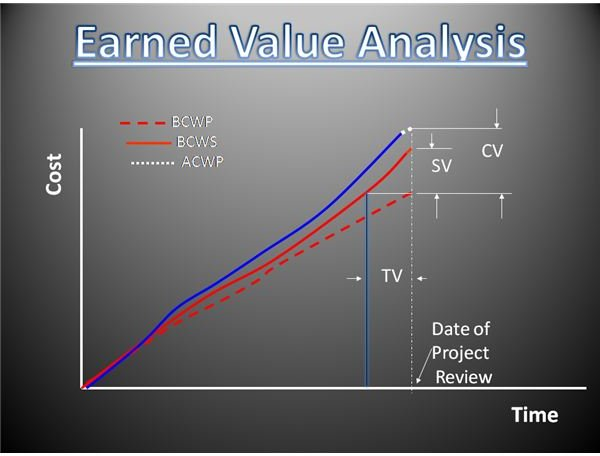What is Earned Value Management? How Can It Be Applied in Projects?

What Is It?

Earned Value Analysis is a project management concept that is used to evaluate the progress of an ongoing project. It combines measurements of cost and time and provides an integrated analysis that results in a very effective tool for project monitoring.

Earned Value Analysis compares the ongoing project's progress against the estimates prepared at the start of the project. It calculates three primary indicators:

• Schedule Variance
• Time Variance
• Cost Variance

Terminology Used in Earned Value Analysis

Earned value analysis is always performed on a specific date and denotes the progress of the project as on that date of review (status date).

Budgeted Cost of Work Scheduled (BCWS): Indicates the cost of work that should have been completed as of the date of review as per the original schedule planned and original budget, i.e. the budgeted (estimated) cost for the work scheduled to be performed until the status date.

Budgeted Cost of Work Performed (BCWP): Indicates the budgeted cost of work that has actually been completed as of the date of review as per the original schedule planned and original budget, i.e. the budgeted (estimated) cost for the actual work performed until the status date.

Actual Cost of Work Performed (ACWP): Indicates the actual cost of work that has been completed as of the date of review (incurred costs and not budgeted costs), i.e. the actual (incurred) cost of the work performed until the status date.

For tasks that have been completed, BCWP and BCWS will be equal. BCWP is a measure of "Earned Value" of a project. Earned Value Analysis can be used to control the cash flow in a project.

Calculating the Variances in Earned Value Analysis

BCWS, BCWP and ACWP are used to calculate the schedule, cost and time variances (see the example) as indicated below.

Schedule Variance (SV) = BCWS – BCWP

Cost Variance (CV) = BCWP – ACWP

Time Variance (TV) = The time required for the project to come back on schedule.

Evaluating the Results of Earned Value Analysis

The above method can be used to calculate the variances (TV, SV, CV) as indicated.

Performance Indexes can also be calculated as given below.

Cost Performance Index (CPI) = BCWP / ACWP

• If it is greater than 1, it indicates the project is progressing under-budget and vice versa.

Schedule Performance Index (SPI) = BCWP / BCWS

• If it is greater than 1, it indicates the project is progressing ahead of schedule and vice versa.

By calculating the 3 variances, CPI and SPI, Earned Value Analysis can be used in Project Monitoring & Control.

A graphical depiction of Earned Value Analysis is shown below.